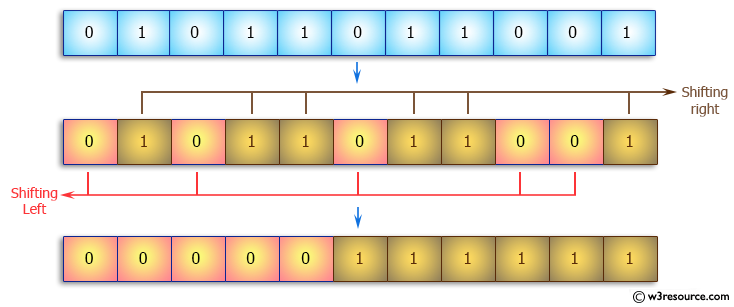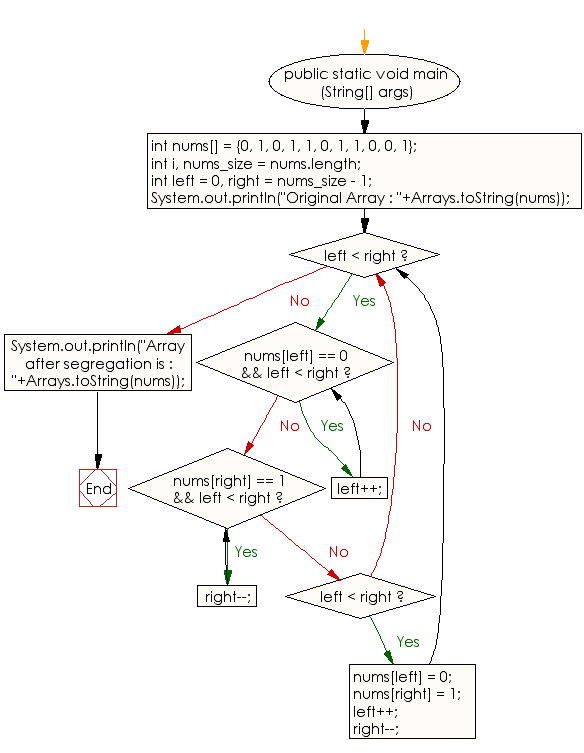﻿ Java: Separate left and right 0s and 1s from an array# Java Array Exercises: Segregate all 0s on left side and all 1s on right side of a given array of 0s and 1s

## Java Array: Exercise-42 with Solution

Write a Java program to segregate all 0s on left side and all 1s on right side of a given array of 0s and 1s.

Pictorial Presentation:Sample Solution:

Java Code:

``````import java.util.*;
import java.lang.*;
public class Main
{
public static void main (String[] args)
{
int nums[] = {0, 1, 0, 1, 1, 0, 1, 1, 0, 0, 1};
int i,  nums_size = nums.length;
int left = 0, right = nums_size - 1;

System.out.println("Original Array : "+Arrays.toString(nums));

while (left < right)
{
/* While  0 at left increment left index  */
while (nums[left] == 0 && left < right)
left++;

/* While we see 1 at right decrement right index*/
while (nums[right] == 1 && left < right)
right--;

if (left < right)
{
nums[left] = 0;
nums[right] = 1;
left++;
right--;
}
}

System.out.println("Array after segregation is : "+Arrays.toString(nums));
}
}
```
```

Sample Output:

```
Original Array : [0, 1, 0, 1, 1, 0, 1, 1, 0, 0, 1]
Array after segregation is : [0, 0, 0, 0, 0, 1, 1, 1, 1, 1, 1]
```

Flowchart:Visualize Java code execution (Python Tutor):

Java Code Editor:

Improve this sample solution and post your code through Disqus

What is the difficulty level of this exercise?

Test your Programming skills with w3resource's quiz.

﻿

## Java: Tips of the Day

countOccurrences

Counts the occurrences of a value in an array.

Use Arrays.stream().filter().count() to count total number of values that equals the specified value.

```public static long countOccurrences(int[] numbers, int value) {
return Arrays.stream(numbers)
.filter(number -> number == value)
.count();
}
```

Ref: https://bit.ly/3kCAgLb